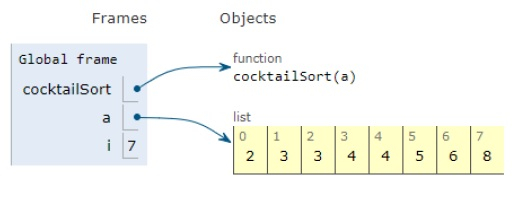# Python Program for Cocktail Sort

PythonServer Side ProgrammingProgramming

Problem statement − We are given a list, we need to perform a bitonic sort on the given list and display the list

Cocktail Sort − Here sort takes place like bubble sort where iteration takes place in both directions.

## Algorithm

Firstly array is traversed from left to right. During traversal, adjacent items are compared and based on the conditions, the values are swapped. By this, the largest number will be at the end of the array.

Now the array is traversed in the opposite direction and based on the conditions, elements are swapped. By this, the smallest number will be at the start.

Now let’s observe the solution in the implementation below −

### Example

Live Demo

# function
def cocktailSort(a):
n = len(a)
flag = True
start = 0
end = n-1
while (flag==True):
# to ignore the result of the previous iteration
flag = False
# left to right traversal
for i in range (start, end):
if (a[i] > a[i+1]) :
a[i], a[i+1]= a[i+1], a[i]
flag=True
# if no swap takes place array remains sorted
if (flag==False):
break
# otherwise, reset the flag
flag = False
# last item is aldready sorted
end = end-1
# iteration from right to left
for i in range(end-1, start-1,-1):
if (a[i] > a[i+1]):
a[i], a[i+1] = a[i+1], a[i]
flag = True
# first element is already sorted
start = start+1
# main
a = [2,5,4,3,8,3,4,6]
cocktailSort(a)
print("Sorted array is:")
for i in range(len(a)):
print (a[i],end=" ")

### Output

Sorted array is:
2 3 3 4 4 5 6 8All the variables are declared in the local scope and their references are seen in the figure above.

## Conclusion

In this article, we have learned about how we can make a Python Program for Cocktail Sort